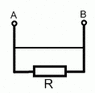# Questions regarding DC circuits

• Engineering
• R A V E N

#### R A V E N

I have one confusing issue to resolve:

What is the resistance between points $$A$$ and $$B$$ in simple system illustrated in attachment?Wouldnt be logical to assume that it is $$0$$,as current strives to flow through path with lowest possible resistance,if I understood it correctly?

Yes,I know that real transmission lines have some resistance,but in network anaylsis their resistance is considered to be equal to zero.

#### Attachments

•ab.gif
985 bytes · Views: 410
Last edited:

I have one confusing issue to resolve:

What is the resistance between points $$A$$ and $$B$$ in simple system illustrated in attachment?Wouldnt be logical to assume that it is $$0$$,as current strives to flow through path with lowest possible resistance,if I understood it correctly?

Yes,I know that real transmission lines have some resistance,but in network anaylsis their resistance is considered to be equal to zero.

You have a short circuit so what ever the resistance of the wire connecting point A and B.

CS

Yeah, it's zero assuming everything's ideal. Mathematically even, you have a wire ($$R=0$$) in parallel with a resistor ($$R=R_0$$). Then the parallel combination is:

$$R_{eq} = \frac{1}{ \frac{1}{0} + \frac{1}{R_0} } = \frac{1}{\infty + \frac{1}{R_0}} = 0$$

I should probably have put some limits in there to be mathematically correct, but you get the idea.

Thanks to both of you!